Get instant live expert help with Excel or Google Sheets“My Excelchat expert helped me in less than 20 minutes, saving me what would have been 5 hours of work!”

#### Post your problem and you’ll get Expert help in seconds.

Your message must be at least 40 characters
Our professional Expert are available now. Your privacy is guaranteed.

# Two-Way Lookup with VLOOKUPRead time: 25 minutes

Excel allows us to lookup with dynamic column number parameter in the VLOOKUP function using MATCH function. This step by step tutorial will assist all levels of Excel users to do the two-way lookup.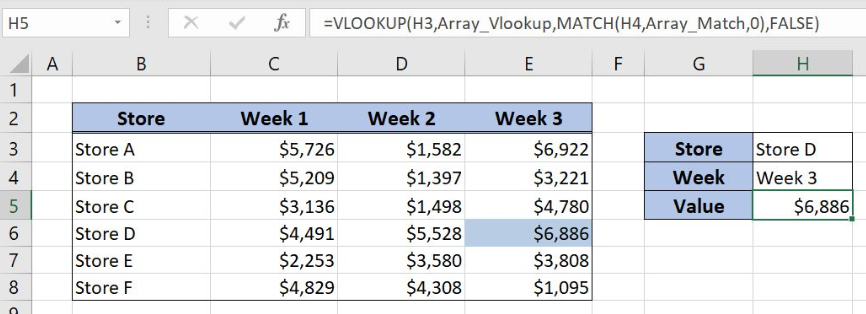Figure 1. Find value per store and week with VLOOKUP and MATCH functions

## Syntax of the VLOOKUP formula

`=VLOOKUP(lookup_value, table_array, col_index_num, range_lookup)`

The parameters of the VLOOKUP function are:

• lookup_value – a value that we want to find in a table_array
• table_array – a range in which we want to lookup
• col_index_num – a column number in table_array from which we would like to get a value
• range_lookup – default value is FALSE. This means that we want to find an exact match for a lookup value.

## Syntax of the MATCH formula

`=MATCH(lookup_value, lookup_array, [match_type])`

The parameters of the MATCH function are:

• lookup_value – a value which we want to find in the lookup_array
• lookup_array – the array where we want to find a value
• [match_type] – a type of match. We put 0 which is an exact match.

## Setting up Our Data to Get the Value Based on the Two Criteria

Our first table consists of 4 columns: “Store” (column B), “Week 1” (column C), “Week 2” (column D) and “Week 3” (column E). The second one has the data for the Store and Week from the first table and an empty cell for the Value. The idea is to get the value from the first table based on the Store and the Week from the second table and to place the result in the cell H5.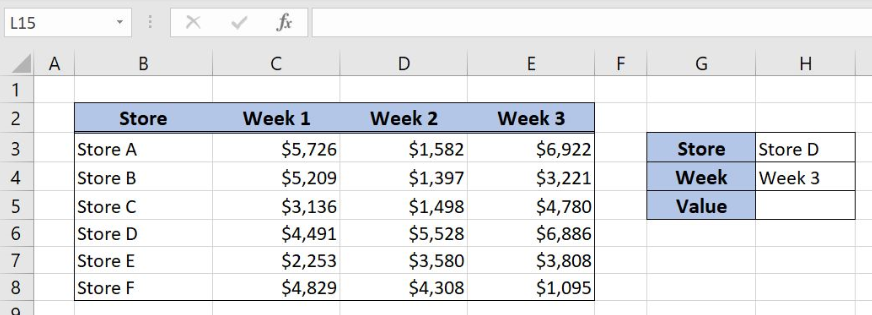Figure 2. Table structure for two-way lookup

## Get the Value Based on Store and Week with VLOOKUP and MATCH Functions

We want to get the value from the first table based on the criteria from the column H for the Store and the Week. In order to make the formula more clear, we will create a named range Array_Vlookup for cell range B3:E8 and a named range Array_Match for the cell range B2:E2.

To create a named range we should follow the steps:

• Select the cell range that should be named
• Click on the name box in Excel
• Write the name for the cell range and press enter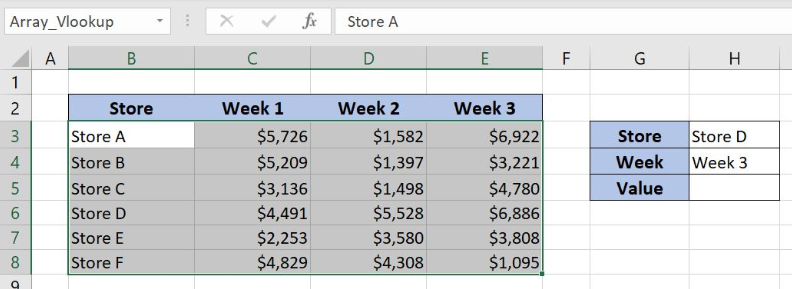Figure 3. Creating a named Array_Vlookup for the VLOOKUP function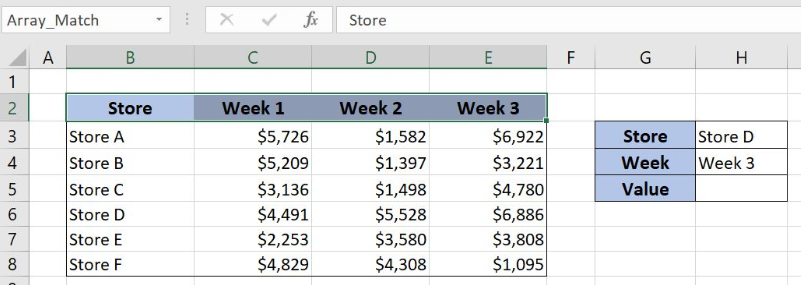Figure 4. Creating a named range Array_Match for the MATCH function

The formula looks like:

`=VLOOKUP(H3,Array_Vlookup,MATCH(H4,Array_Match,0),FALSE)`

The lookup_value is the cell H3 “Store D” while the table_array is the named range Array_Vlookup. The parameter col_index_num is the formula `MATCH(H4,Array_Match,0)` while the range_lookup is FALSE as we want the exact match.

The parameter lookup_value in the MATCH function is the cell H4 “Week 3” while the lookup_array is the named range Array_Match. The match_type is 0 since we want the exact match.

To apply the formula we need to follow these steps:

• Select cell H5 and click on it
• Insert the formula: `=VLOOKUP(H3,Array_Vlookup,MATCH(H4,Array_Match,0),FALSE)`
• Press enter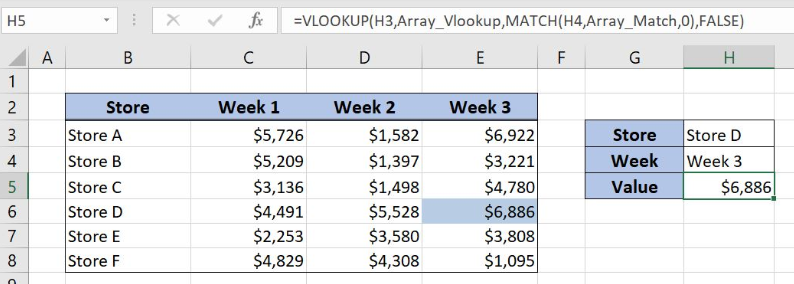Figure 5. Two-way lookup with VLOOKUP and MATCH functions

The MATCH function gives us information about the col_index_num parameter of the VLOOKUP function. The result of the MATCH function is number 4 because “Week 3” is in the 4th position in the range Array_Match.

The VLOOKUP function gets the value from the Array_Vlookup with the lookup_value “Store D” and column number 4. Final formula result is the number \$6,886, value for the “Store D” from the “Week 3”.

Most of the time, the problem you will need to solve will be more complex than a simple application of a formula or function. If you want to save hours of research and frustration, try our live Excelchat service! Our Excel Experts are available 24/7 to answer any Excel question you may have. We guarantee a connection within 30 seconds and a customized solution within 20 minutes.

Solution examplesI need a formula to compare the data in two columns and then export the mismatched data in the 3rd column
Solved by S. Q. in 20 minsI filtered data in column A (it is labeled ID #) of a data set. On my next worksheet, column A is also the ID #, but it is a different data set. I want to filter the ID #'s the same for the two sheets, but how do I do that being that I am working with two different sets of data?
Solved by V. H. in 22 minsI'm looking for a formula or format for if there is data in c4 on a sheet... It adds extra information underneath
Solved by X. E. in 20 minshi, my vlookup wont work in some rows but does in others. its temperemental. e.g it will work on rows 10 to 15, then not row 16 and 17, but the will for the rest.
Solved by K. Q. in 21 minsI have a pivot table in which per order (on the rows) stands how much products they ordered per size (on the columns). I want to determine which combinations of quantities of sizes people order. And I want to count these combinations.
Solved by E. W. in 60 mins